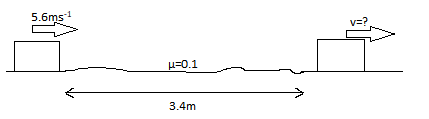# A box of unknown mass is sliding with an initial speed v_{i}=5.6 m/s across a horizontal...

## Question:

A box of unknown mass is sliding with an initial speed {eq}v_{i}=5.6 m/s {/eq}across a horizontal frictionless warehouse floor when it encounters a rough section of flooring d = 3.40 m long. The coefficient of kinetic friction between the rough section of flooring and the box is 0.100. Using energy considerations, determine the final speed of the box after sliding across the rough section of flooring.

## Work Done by Frictional Force:

There are two ways of solving this problem.

1. Assuming the mass of the block and following energy conservation laws.
2. Transforming this problem into a simple kinematic problem and solving for the final velocity.

Actually, both methods are quite similar. They only differ by a constant i.e. mass of the block.

In method 1, one can assume the mass of the block and write the initial kinetic energy of the block. This initial energy can be equated to the sum of energy dissipated by the frictional force and the final kinetic energy. From here, the final velocity can be calculated.

In the second method, the block can be thought of as a particle moving with some initial velocity. When the block is subjected to some deceleration(obstruction) the final velocity can be calculated with the basic equations of motion.This problem can be thought of as a basic kinematic problem, where a particle moving with uniform velocity is subjected to a certain deceleration for some distance. Now we need to find the final velocity of the body.

The deceleration due to the rough floor will be

{eq}a=-\mu g=-0.1\times 9.8=-0.98\:ms^{-2} {/eq}

Using the third equation of motion,

{eq}v^{2}=u^{2}+2as {/eq}

we can calculate the new velocity after the block passes the rough surface.

{eq}\therefore v^{2}=5.6^{2}+2(-0.98)(3.40)=24.696 \therefore v=\sqrt{24.696}=4.96950\:ms^{-1} {/eq}